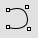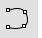# CurveCurve

Free-Form >

Control Points

The Curve command draws a curve from control point locations.Curve control points match input.

#### Steps

1. Pick the start of the curve.
2. Pick the next points.
3. Press Enter to end the curve.
The Curve command makes a curve of degree <number of points> -1 as long as the number of control points is less than or equal to the degree setting.

Command-line options

##### AutoClose

Closes the curve when the cursor moves close to the curve's start point.

#### AutoClose steps

1. Move the cursor close to the start point of the curve, and pick.
The curve will close.
2. Press the Alt key to suspend automatic closing.

##### Degree

Specifies the degree of the curve.

The resulting curve will not be the degree you request unless the control point number is at least one more than the degree number.

##### PersistentClose

Closes the curve as soon as there are two points placed.

##### Close

Closes the curve smoothly, creating a periodic curve.

##### Sharp

Closes the curve with a kink, creating a non-periodic curve.

##### Undo

Reverses the last action.

# InterpCrvCurve

Free-Form >

Interpolate Points

The InterpCrv command draws a curve through selected locations in space.

Many CAD programs use the term spline to describe an interpolated curve.Curve matches input.

#### Steps

1. Pick the start of the curve.
2. Pick the next points.
3. Press Enter to end the curve.

Command-line options

##### AutoClose

Closes the curve when the cursor moves close to the curve's start point.

#### Steps

1. Move the cursor close to the start point of the curve, and pick.
The curve will close.
2. Press the Alt key to suspend automatic closing.

##### Degree

Specifies the degree of the curve.

Unlike the Curve command, the resulting curve is always the degree you request even if only two points (start and end) are picked.

##### PersistentClose

Closes the curve as soon as there are two points placed.

If you continue to pick points, the curve updates the shape while remaining closed.

##### Knots

Determines how an interpolated curve is parameterized. When the spacing between the picked points is equal for the whole curve, all three parameterizations generate the same curve.

When you draw an interpolated curve, the points you pick are converted into knot values on the curve. The parameterization means how the intervals between knots are chosen.

##### Uniform

The knot spacing is always 1 and is not based on the physical spacing of the points. Uniform parameterization can be used if the point spacing is roughly equal and it is desirable to draw several curves that have the same parameterization. This is possible only with uniform curves (unless you rebuild the curves). Uniform guarantees that every control point affects the surface exactly the same way, no matter how much the surface is edited.

##### Chord

The spacing between the picked points is used for the knot spacing. This makes curves that have widely varying point spacing behave better than uniform curves.

##### SqrtChrd

The square root of the spacing between picked points is used for the knot spacing.

##### StartTangent

Draws the start of curve tangent to another curve or tangent to a direction shown by picking a start and end direction.

##### EndTangent

Draws the end of the curve tangent to another curve or tangent to a direction shown by picking a start and end direction.

##### Close

Closes the curve smoothly, creating a periodic curve

##### Sharp

Closes the curve with a kink, creating a non-periodic curve.

##### Undo

Reverses the last action.